2019-12-05 13:35:26 weixin_44223883 阅读数 127
• ###### 定时器和计数器-第1季第10部分

本课程是《朱有鹏老师单片机完全学习系列课程》第1季第10个课程，主要内容是51单片机的定时器和计数器，本课程的学习目标是对定时器的作用和意义有深入理解，掌握通过操作寄存器来操作硬件的思路和方法。

1964 人正在学习 去看看 朱有鹏

## STM32单片机定时器1设置成为AB项编码器模式

``````void TIM1_Init()
{
TIM_TimeBaseInitTypeDef TIM_TimeBaseStructure;
TIM_ICInitTypeDef TIM_ICInitStructure;
GPIO_InitTypeDef GPIO_InitStructure;

RCC_APB2PeriphClockCmd(RCC_APB2Periph_TIM1, ENABLE);//
RCC_APB2PeriphClockCmd(RCC_APB2Periph_GPIOA, ENABLE);//

GPIO_InitStructure.GPIO_Pin = GPIO_Pin_8|GPIO_Pin_9;
GPIO_InitStructure.GPIO_Mode = GPIO_Mode_IN_FLOATING;
GPIO_Init(GPIOA, &GPIO_InitStructure);
TIM_TimeBaseStructInit(&TIM_TimeBaseStructure);
TIM_TimeBaseStructure.TIM_Prescaler = 0x0; //
TIM_TimeBaseStructure.TIM_Period = ENCODER_TIM_PERIOD;
TIM_TimeBaseStructure.TIM_ClockDivision = TIM_CKD_DIV1;
TIM_TimeBaseStructure.TIM_CounterMode = TIM_CounterMode_Up;
TIM_TimeBaseStructure.TIM_RepetitionCounter = 0;//TIM1高级定时器特有
TIM_TimeBaseInit(TIM1, &TIM_TimeBaseStructure);
TIM_EncoderInterfaceConfig(TIM1, TIM_EncoderMode_TI12, TIM_ICPolarity_Rising, TIM_ICPolarity_Rising);
TIM_ICStructInit(&TIM_ICInitStructure);
TIM_ICInitStructure.TIM_ICFilter = 10;
TIM_ICInit(TIM1, &TIM_ICInitStructure);

TIM_ClearFlag(TIM1, TIM_FLAG_Update);
TIM_ITConfig(TIM1, TIM_IT_Update, ENABLE);

TIM_SetCounter(TIM1,0);
TIM_Cmd(TIM1, ENABLE);
}
``````

## 使用TIM8进行编码器值采集

``````void TIM8_Init(u16 arr,u16 psc)
{
TIM_TimeBaseInitTypeDef  TIM_TimeBaseStructure;
NVIC_InitTypeDef NVIC_InitStructure;

RCC_APB2PeriphClockCmd(RCC_APB2Periph_TIM8, ENABLE); //时钟使能

TIM_TimeBaseStructure.TIM_Period = arr; //设置自动重装载寄存器周期值
TIM_TimeBaseStructure.TIM_Prescaler =psc;//设置预分频值
TIM_TimeBaseStructure.TIM_ClockDivision = 0; //设置时钟分割
TIM_TimeBaseStructure.TIM_CounterMode = TIM_CounterMode_Up;//向上计数模式
TIM_TimeBaseStructure.TIM_RepetitionCounter = 0;//重复计数设置
TIM_TimeBaseInit(TIM8, &TIM_TimeBaseStructure); //参数初始化
TIM_ClearFlag(TIM8, TIM_FLAG_Update);//清中断标志位

TIM_ITConfig(      //使能或者失能指定的TIM中断
TIM8,            //TIM1
TIM_IT_Update  | //TIM 更新中断源
TIM_IT_Trigger,  //TIM 触发中断源
ENABLE  	     //使能
);

//设置优先级
NVIC_InitStructure.NVIC_IRQChannel = TIM8_UP_IRQn;
NVIC_InitStructure.NVIC_IRQChannelPreemptionPriority = 0;//先占优先级0级
NVIC_InitStructure.NVIC_IRQChannelSubPriority = 0;  	   //从优先级0级
NVIC_InitStructure.NVIC_IRQChannelCmd = ENABLE;
NVIC_Init(&NVIC_InitStructure);
TIM_Cmd(TIM8, ENABLE);  //使能TIMx外设

}
``````

## TIM8中断服务函数进行编码器值累计求和

``````void TIM8_UP_IRQHandler(void)
{
if (TIM_GetITStatus(TIM8, TIM_IT_Update) != RESET)//检查指定的TIM中断发生与否:TIM 中断源
{
TIM_ClearITPendingBit(TIM8, TIM_IT_Update);//清除TIMx的中断待处理位:TIM 中断源

Encoder1=(short)TIM1->CNT;
Encoder2=(short)TIM2->CNT;
Encoder3=(short)TIM3->CNT;
Encoder4=(short)TIM4->CNT;

//加上'0'转换成为字符型

//四路电机编码值
MasterToSlvaeData=Encoder1+'0';
MasterToSlvaeData=Encoder2+'0';
MasterToSlvaeData=Encoder3+'0';
MasterToSlvaeData=Encoder4+'0';

//小车偏航角
MasterToSlvaeData=YawToSlvae+'0';

SendDataToSlave(USART3,MasterToSlvaeData,0,5);

TIM1->CNT=0;
TIM2->CNT=0;
TIM3->CNT=0;
TIM4->CNT=0;

}
}
``````

2019-06-08 23:58:02 weixin_44895651 阅读数 2121
• ###### 定时器和计数器-第1季第10部分

本课程是《朱有鹏老师单片机完全学习系列课程》第1季第10个课程，主要内容是51单片机的定时器和计数器，本课程的学习目标是对定时器的作用和意义有深入理解，掌握通过操作寄存器来操作硬件的思路和方法。

1964 人正在学习 去看看 朱有鹏

## 51定时器中断

#### 1.定时器/计数器

51单片机有2个16位定时器/计数器：定时器0（T0为P3.4） 和定时器1（T1为P3.5）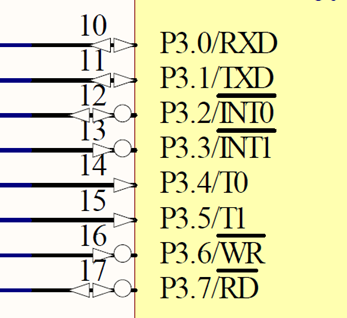#### 使用51定时/计数器步骤：

（1）启动定时/计数器（通过TCON控制器）
（2）设置定时/计数器工作模式（通过TMOD控制器）
（3）查询定时/计数器是否溢出（读TCON内TF位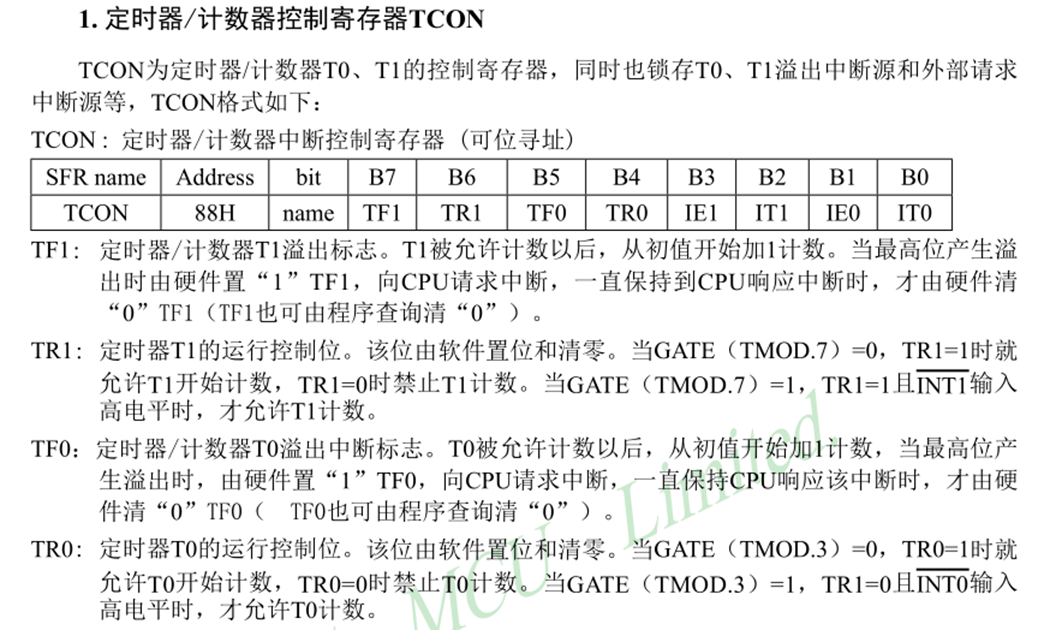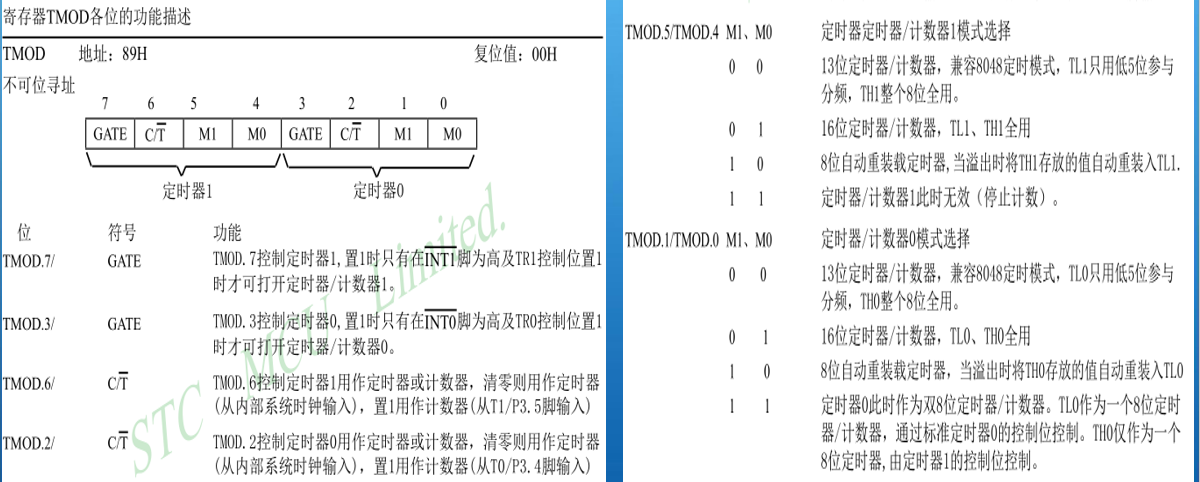#### 3.代码片：

（1）定时器定时：

``````#include <reg52.h>
#include <intrins.h>
#define uint unsigned int
#define uchar unsigned char
sbit DU = P2^6;
sbit WE = P2^7;
uchar  code tabel[]= {0x3F, 0x06, 0x5B, 0x4F, 0x66, 0x6D, 0x7D, 0x07, 0x7F, 0x6F,};
void delay(uint z)
{
uint x,y;
for(x = z; x > 0; x--)
for(y = 114; y > 0 ; y--);
}
void display(uchar i)
{
uchar bai, shi, ge;
bai = i / 100; //236 / 100  = 2
shi = i % 100 / 10; //236 % 100 / 10 = 3
ge  = i % 10;//236 % 10 =6
P0 = 0XFF;
WE = 1;
P0 = 0XFE; //1111 1110
WE = 0;
DU = 1;
P0 = tabel[bai];//
DU = 0;
delay(5);
P0 = 0XFF;
WE = 1;
P0 = 0XFD; //1111 1101
WE = 0;
DU = 1;
P0 = tabel[shi];//
DU = 0;
delay(5);
P0 = 0XFF;
WE = 1;
P0 = 0XFB; //1111 1011
WE = 0;
DU = 1;
P0 = tabel[ge];//
DU = 0;
delay(5);
}
void timer0Init()
{
TR0 = 1;
TMOD = 0X01;
TH0 = 0x4b;
TL0 = 0xfd;
}
void main()
{
uchar mSec, Sec;
timer0Init();
while(1)
{
if(TF0 == 1)
{
TF0 = 0;
TH0 = 0x4b;
TL0 = 0xfd;
mSec++;
if(mSec == 20)
{
mSec = 0;
Sec++;
}
}
display(Sec);
if(Sec > 10)
Sec = 0;
}
}
``````

（2）定时器计数：

``````#include <reg52.h>
#include <intrins.h>
#define uint unsigned int
#define uchar unsigned char
sbit DU = P2^6;
sbit WE = P2^7;
sbit LED1 = P1^0;
uchar  code tabel[]= {0x3F, 0x06, 0x5B, 0x4F, 0x66, 0x6D, 0x7D, 0x07, 0x7F, 0x6F,};
void delay(uint z)
{
uint x,y;
for(x = z; x > 0; x--)
for(y = 114; y > 0 ; y--);
}
void display(uchar i)
{
uchar bai, shi, ge;
bai = i / 100;
shi = i % 100 / 10;
ge  = i % 10;
P0 = 0XFF;
WE = 1;
P0 = 0XFE; //1111 1110
WE = 0;
DU = 1;
P0 = tabel[bai];//
DU = 0;
delay(5);
P0 = 0XFF;
WE = 1;
P0 = 0XFD;
WE = 0;
DU = 1;
P0 = tabel[shi];
DU = 0;
delay(5);
P0 = 0XFF;
WE = 1;
P0 = 0XFB; //1111 1011
WE = 0;
DU = 1;
P0 = tabel[ge];//
DU = 0;
delay(5);
}
void timer0Init()
{
TR0 = 1;
TMOD |= 0X05;
TH0 = 0;
TL0 = 0;
}
void timer1Init()
{
TR1 = 1;
TMOD |= 0X10;
TH1 = 0x4b;
TL1 = 0xfd;
}
void main()
{
uchar mSec, Sec;
timer0Init();
timer1Init();
while(1)
{
if(TF1 == 1)
{
TF1 = 0;
TH1 = 0x4b;
TL1 = 0xfd;
mSec++;
if(mSec == 10)
{
mSec = 0;
LED1 = ~LED1;
}
}
display(TL0);
}
}
``````

（3）定时器中断：

``````#include <reg52.h>
#include <intrins.h>
#define uint unsigned int
#define uchar unsigned char
sbit DU = P2^6;
sbit WE = P2^7;
sbit key_s2 = P3^0;
sbit key_s3 = P3^1;
uchar num;
uchar mSec, Sec;
uchar code SMGduan[]= {0x3F, 0x06, 0x5B, 0x4F, 0x66, 0x6D, 0x7D, 0x07, 0x7F, 0x6F,};
uchar code SMGwei[] = {0xfe, 0xfd, 0xfb};
void delay(uint z)
{
uint x,y;
for(x = z; x > 0; x--)
for(y = 114; y > 0 ; y--);
}
void display(uchar i)
{
static uchar wei;
P0 = 0XFF;
WE = 1;
P0 = SMGwei[wei];
WE = 0;
switch(wei)
{
case 0: DU = 1; P0 = SMGduan[i / 100]; DU = 0; break;
case 1: DU = 1; P0 = SMGduan[i % 100 / 10]; DU = 0; break;
case 2: DU = 1; P0 = SMGduan[i % 10]; DU = 0; break;
}
wei++;
if(wei == 3)
wei = 0;
}
void timer0Init()
{
EA = 1;
ET0 = 1;
TR0 = 1;
TMOD = 0X01;
TH0 = 0xED;
TL0 = 0xFF;
}
void main()
{
timer0Init();
while(1)
{
if(key_s2 == 0)
{
delay(20);
if(key_s2 == 0)
{
if(num != 120)
num++;
while(!key_s2);
}
}
if(key_s3 == 0)
{
delay(20);
if(key_s3 == 0)
{
if(num > 0)
num--;
while(!key_s3);
}
}
}
}
void timer0() interrupt 1
{
TH0 = 0xED;
TL0 = 0xFF;
display(num);
}
``````

2010-07-17 15:10:00 feiyinzilgd 阅读数 5415
• ###### 定时器和计数器-第1季第10部分

本课程是《朱有鹏老师单片机完全学习系列课程》第1季第10个课程，主要内容是51单片机的定时器和计数器，本课程的学习目标是对定时器的作用和意义有深入理解，掌握通过操作寄存器来操作硬件的思路和方法。

1964 人正在学习 去看看 朱有鹏

msp430 定时器学习笔记

_BIS_SR(LPM2_bits + GIE)  //进入LPM3低功耗模式和开总中断允许

#pragma  vector=TIMERA0_VECTOR
__interrupt void Timer_A(void)
{

}

TACTL   timerA控制寄存器
TAR     timerA计数器
CCTL0   捕获/比较控制寄存器0
CCRO    捕获/比寄存器0(具有最高中断优先级别，单独使用一个中断向量)
CCTL1   捕获/比较寄存器1
CCR1    捕获/比较寄存器1
CCTL2   捕获/比较寄存器2
CCR2    捕获/比较寄存器2
TAIV    中断向量寄存器

TimerA输出模式：

TACTL
15-10 9 8 7 6 5 4 3 2 1 0

SSEL1    SSEL0
0          0  (特定外部信号)
0          1  (TASSEL0)  ACLK
1          0  (TASSEL1)  SMCLK
1          1  (INCLK)

0  0  停止模式。停止模式下，定时器不复位，回复工作后，按照停止时刻的状态继续运行

0  1  增计数模式
TAR == CCR1的时候产生一次中断。但是只有TAR == CCR0的时候，定时器才复位。（65535）

1  0  连续技术模式

0---0x0FFFH之后重新计数
1  1  增/减计数模式   先增，后减，产生堆成波形。

1，此时时钟处于高，则遇到下降沿立马复位。

2.如果时钟处于低，则在下一个时钟周期下降沿复位(多往前走一个时钟周期)

//就是把当前计数器TAR的值抓取放到CCRX当中

2019-03-04 20:04:04 imxlw00 阅读数 112
• ###### 定时器和计数器-第1季第10部分

本课程是《朱有鹏老师单片机完全学习系列课程》第1季第10个课程，主要内容是51单片机的定时器和计数器，本课程的学习目标是对定时器的作用和意义有深入理解，掌握通过操作寄存器来操作硬件的思路和方法。

1964 人正在学习 去看看 朱有鹏

### 定时器的寄存器

T0和T1的控制由两个8位特殊功能寄存器完成：一个为定时器的方式选择寄存器TMOD用于确定定时器还是计数器工作模式；另一个叫做定时器控制寄存器TCON，可以决定定时器或计数器的启动、停止及进行中断控制。

• TCON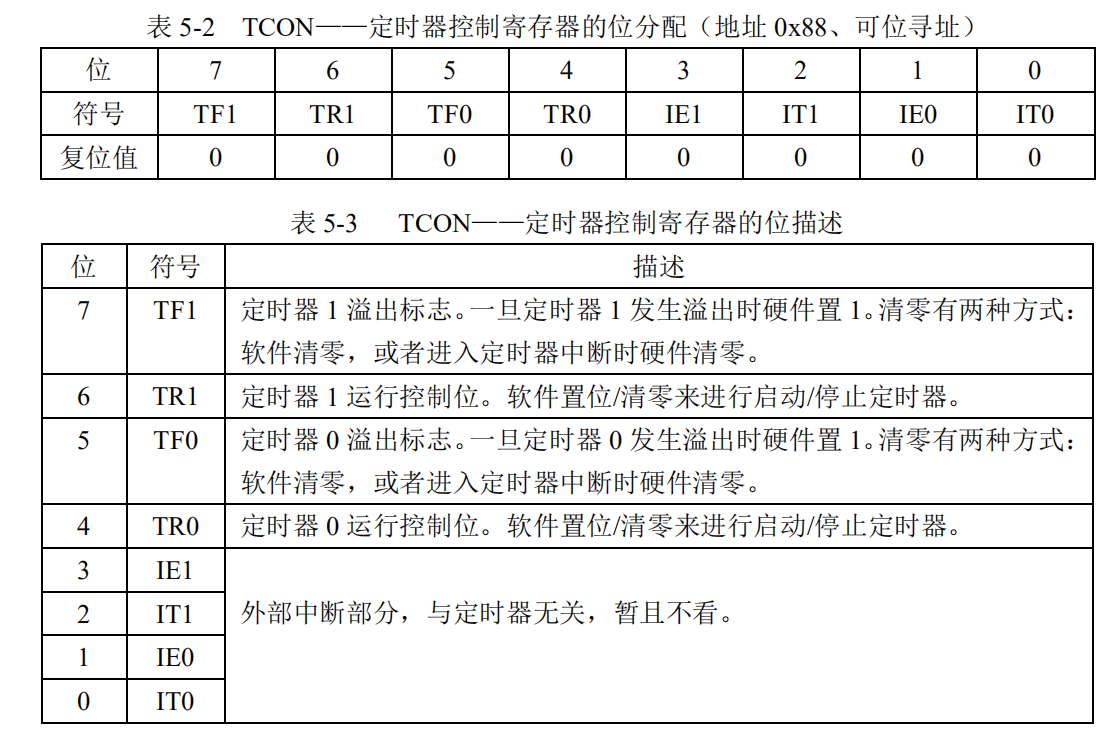• TMOD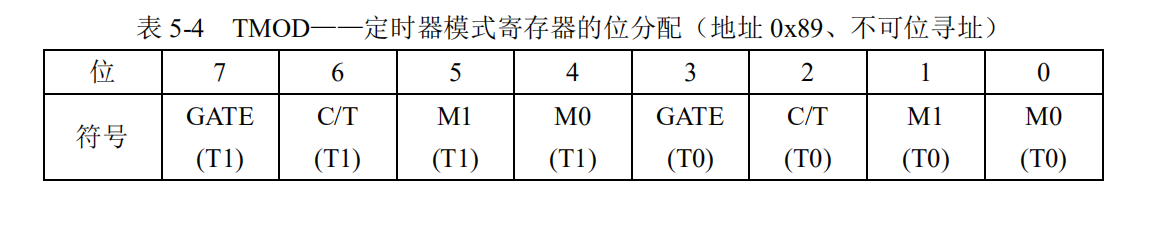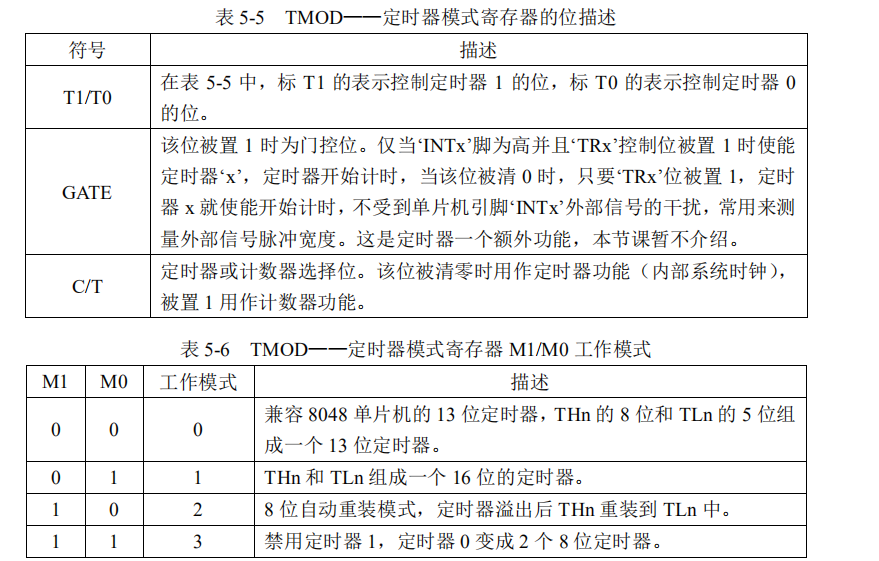模式 1，是 THn 和 TLn 组成了一个 16 位的定时器，计数范围是 0～65535。
模式 2，是 8 位自动重装载模式，计数范围 0～255。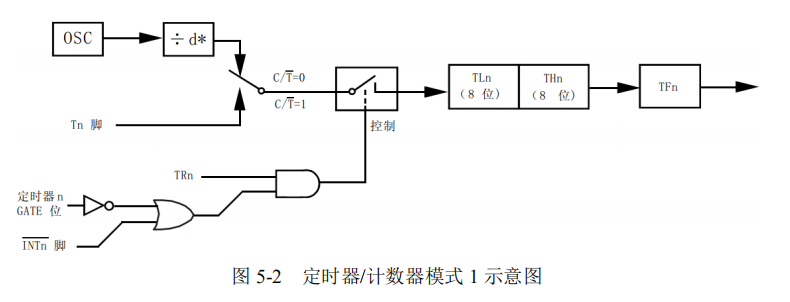### 定时器的应用

``````#include <reg52.h>

sbit LED = P0^0;
sbit ENLED = P1^4;

void main()
{
unsigned char cnt = 0;  //定义一个计数变量，记录T0溢出次数

ENLED = 0;    //使能U3，选择独立LED
TMOD = 0x01;  //设置T0为模式1
TH0  = 0xB8;  //为T0赋初值0xB800
TL0  = 0x00;
TR0  = 1;     //启动T0

while (1)
{
if (TF0 == 1)        //判断T0是否溢出
{
TF0 = 0;         //T0溢出后，清零中断标志
TH0 = 0xB8;      //并重新赋初值
TL0 = 0x00;
cnt++;           //计数值自加1
if (cnt >= 50)   //判断T0溢出是否达到50次
{
cnt = 0;     //达到50次后计数值清零
LED = ~LED;  //LED取反：0-->1、1-->0
}
}
}
}
``````

2020-01-15 12:52:34 qq_44981039 阅读数 33
• ###### 定时器和计数器-第1季第10部分

本课程是《朱有鹏老师单片机完全学习系列课程》第1季第10个课程，主要内容是51单片机的定时器和计数器，本课程的学习目标是对定时器的作用和意义有深入理解，掌握通过操作寄存器来操作硬件的思路和方法。

1964 人正在学习 去看看 朱有鹏

### 定时/计数器

51单片机有T0和T1两个定时器，定时器0有四种工作方式：方式0:13位定时 /计数器模式方式1:16位定时 /计数器模式方式2:8位可重装载定时 /计数器模式方式3：两个单独8位定时 /计数器模式定时器1只有三种方式，同上面三种。

①使用软件定时 优点：可随意修改定时时间，一次编写，多次调用 缺点占用CPU时间，降低CPU的利用率。
②时基电路 优点：使用方便，缺点：硬件电路连接好之后，定时时间不好改变。利用时间计算电阻和电容的值，延时可能会出现误差
③可编程芯片定时：优点：定时准确，可修改时间，使用灵活，可与单片机扩展连接。缺点：电路连接较不方便。

7 6 5 4 3 2 1 0

①选择工作步骤
②选择控制方式
③选择定时器模式
④定时器赋初值
⑤开中断
⑥开总中断
⑦开定时器

X：定时时间

### 具体实现代码与电路

###### 代码
``````//定时器，led闪烁，
#include<reg51.h>
sbit  led=P1^0;
void time();
void main()
{
led=1;
time();
while(1);
}
void time()  //定时器设置
{
TMOD=0x10; // 定时器0，工作方式1
TH0=0x00;
TL0=0x00;
EA=1;  //开总中断
ET0=1;  //开定时器中断
TR0=1;   //启动定时器
}
void timer()interrupt  1
{
TH0=0x00;
TL0=0x00;
led=~led;
}``````
###### 电路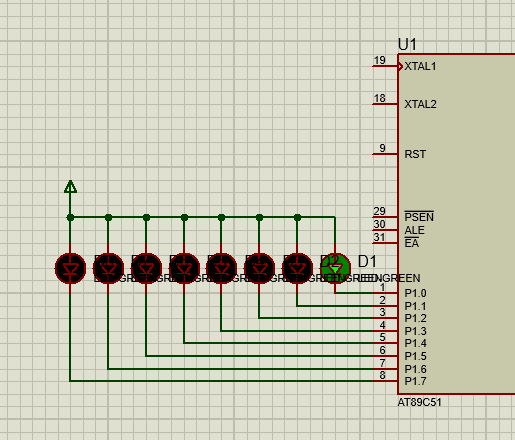###### 优化闪烁周期定时500ms
``````//led闪烁500ms
#include<reg51.h>
sbit  led=P1^0;
int i=0;
void time();
void main()
{
led=1;
time();
while(1)
{
if(i==10)
{
led=~led;
i=0;
}
}
}
void time()
{
TMOD=0x10;
TH0=0x4c; //50ms
TL0=0x00;
EA=1;
ET1=1;
TR1=1;
}
void timer()interrupt  3
{
TH0=0x4c;
TL0=0x00;
i++;
}``````